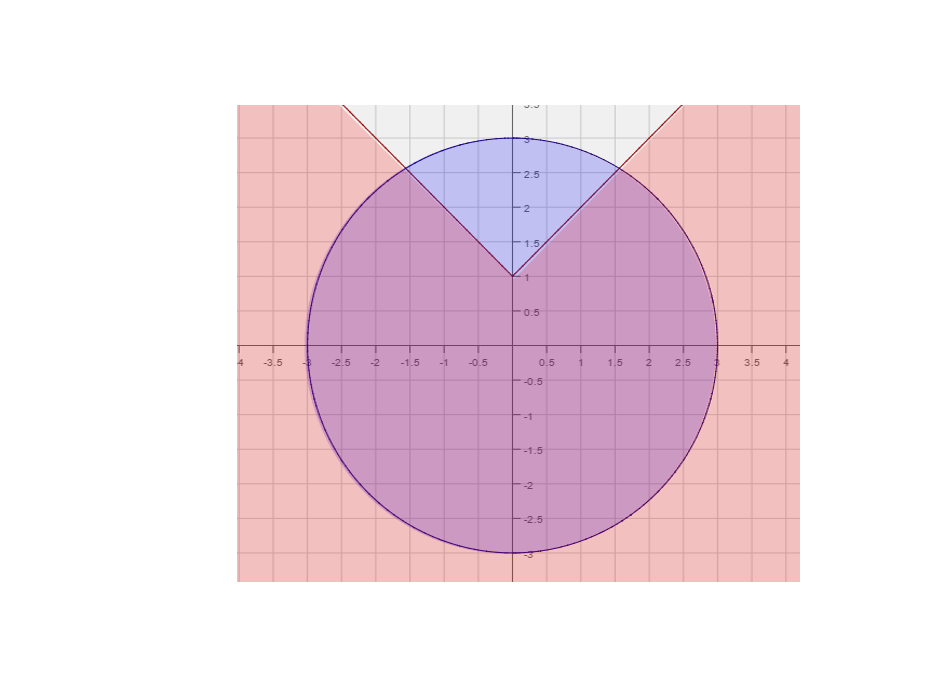# Difference between revisions of "005 Sample Final A, Question 19"

Question Consider the following function,

$f(x)=-\sin \left(3x+{\frac {\pi }{2}}\right)+1$a. What is the amplitude?
b. What is the period?
c. What is the phase shift?
d. What is the vertical shift?
e. Graph one cycle of f(x). Make sure to label five key points.

Foundations:
1) For parts (a) - (d), How do we read the relevant information off of $A\sin(Bx+C)+D?$2) What are the five key points when looking at $\sin(x)?$1) The amplitude is A, the period is ${\frac {2\pi }{B}}$, the horizontal shift is left by C units if C is positive and right by C units if C is negative, the vertical shift is up by D if D is positive and down by D units if D is negative.
2) The five key points are $(0,0),~({\frac {pi}{2}},1),~(\pi ,0),~({\frac {3\pi }{2}},0),~{\text{and }}(2\pi ,0).$Solution:

Step 1:
Amplitude: -1, period: ${\frac {2\pi }{3}}~$, phase shift: Left by ${\frac {\pi }{2}}~$and vertical shift up by 1.

Step 2:
Now that we have graphed both functions we need to know which region to shade with respect to each graph.
To do this we pick a point an equation and a point not on the graph of that equation. We then check if the
point satisfies the inequality or not. For both equations we will pick the origin.
$y<\vert x\vert +1:$Plugging in the origin we get, $0<\vert 0\vert +1=1$. Since the inequality is satisfied shade the side of
$y<\vert x\vert +1$that includes the origin. We make the graph of $y<\vert x\vert +1$, since the inequality is strict.
$x^{2}+y^{2}\leq 9:$$(0)^{2}+(0)^{2}=0\leq 9$. Once again the inequality is satisfied. So we shade the inside of the circle.
We also shade the boundary of the circle since the inequality is $\leq$The final solution is the portion of the graph that below $y=\vert x\vert +1$and inside $x^{2}+y^{2}=9$